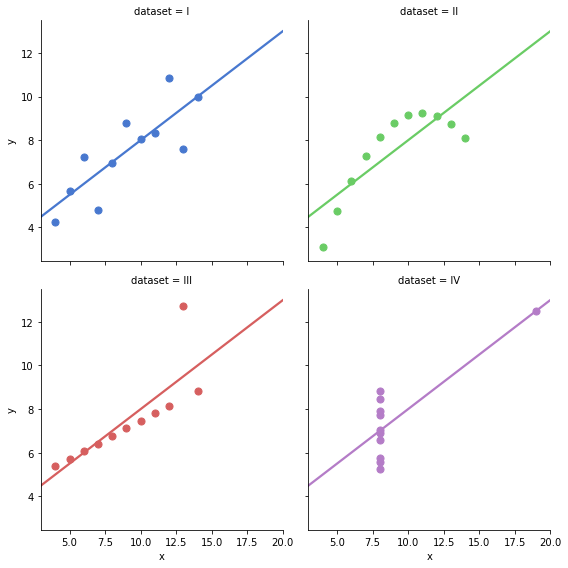# Anscombe's Quartet

Friday, February 2, 2018

We often look for summary statistics during EDA (Exploratory Data Analysis). But, sometimes these statistics may give us wrong interpretation of the data. In 1973, a statistician Francis Anscombe demonstrated it with the help of four datasets known as Anscombe’s quartet.

Now, let’s look at the statistical summary:

All the datasets have the same statistical summary: mean, standard deviation, same correlation between x and y (3 decimal places). Now, let’s visualize the datasets:OMG, these datasets are so much different while they seemed the same by looking at the statistical summary. Now, we realize the importance of graphing data before analyzing it.
Hence, visualization is a crucial and integral part of exploratory data analysis.

References: